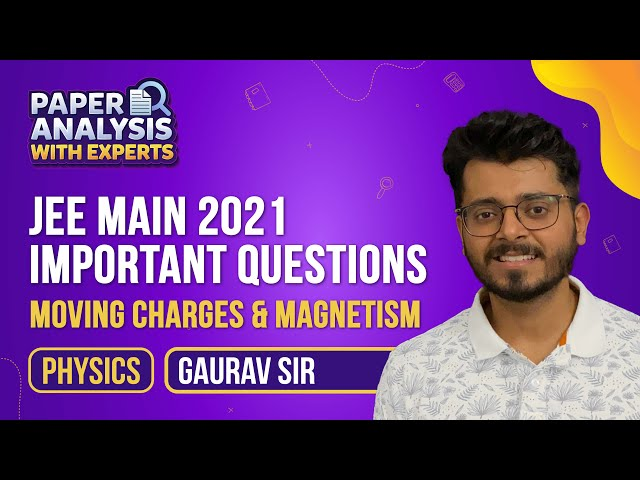# Magnetic Force

Magnetic force is a consequence of electromagnetic force and is caused due to the motion of charges. We have learned that a moving charge surrounds itself with a magnetic field. With this context, the magnetic force can be described as a force that arises due to interacting magnetic fields. Learn more about magnetic force in detail.

## What Is Magnetic Force?

If we place a point charge q in the presence of both a magnitude field given by magnitude B(r) and an electric field given by a magnitude E(r), then the total force on the electric charge q can be written as the sum of the electric force and the magnetic force acting on the object (Felectric Fmagnetic ).

Magnetic force can be defined as:

The magnetic force between two moving charges may be described as the effect exerted upon either charge by a magnetic field created by the other.

### How To Find Magnetic Force?

The magnitude of the magnetic force depends on how much charge is in how much motion in each of the objects and how far apart they are.

Mathematically, we can write magnetic force as:

 $$\begin{array}{l}F=q[E(r)+v\times B(r)]\end{array}$$

This force is termed as the Lorentz Force. It is the combination of the electric and magnetic force on a point charge due to electromagnetic fields. The interaction between the electric field and the magnetic field has the following features:

• The magnetic force depends upon the charge of the particle, the velocity of the particle and the magnetic field in which it is placed. The direction of the magnetic force is opposite to that of a positive charge.
• The magnitude of the force is calculated by the cross product of velocity and the magnetic field, given by q [ v × B ]. The resultant force is thus perpendicular to the direction of the velocity and the magnetic field, the direction of the magnetic field is predicted by the right-hand thumb rule.
• In the case of static charges, the total magnetic force is zero.

## Watch the Video and Learn about Force on a Moving Charge in Magnetic Field## Magnetic Force on a Current-Carrying Conductor

Let us now discuss the force due to the magnetic field in a straight current-carrying rod.

• We consider a rod of uniform length l and cross-sectional area A.
• In the conducting rod, let the number density of mobile electrons be given by n.

Then the total number of charge carriers can be given by nAI, where I is the steady current in the rod. The drift velocity of each mobile carrier is assumed to be given as vd. When the conducting rod is placed in an external magnetic field of magnitude B, the force applied on the mobile charges or the electrons can be given as:

 $$\begin{array}{l}F=(nAI)qvd\times B\end{array}$$

Where q is the value of charge on the mobile carrier.

As nqvd is also the current density j and A×|nqvd| is the current I through the conductor, then we can write:

 $$\begin{array}{l}F=[(nqevd)AI]\times B=[jAI]\times B=Il\times B\end{array}$$

Where I is the vector of magnitude equal to the length of the conducting rod.

## Moving Charges and Magnetism Lecture in Hindi## Solved Examples

Q1. The direction of the current in a copper wire carrying a current of 6.00 A through a uniform magnetic field with a magnitude of 2.20T is from the left to the right of the screen. The direction of the magnetic field is upward-left, at an angle of θ = 3π/4 radians from the current direction. Determine the magnitude and direction of the magnetic force acting on a 0.100 m section of the wire.

Solution:

The magnitude of the magnetic force can be found using the formula:

$$\begin{array}{l}\vec{F}{\rightarrow}=ILB\sin \theta \widehat{n}\end{array}$$

where,
F is the magnetic vector (N)
I is the current magnitude (A)
L is the length vector (m)
L is the length of the wire (m)
B is the magnetic field vector (T)
B is the magnetic field magnitude (T)
θ is the angle between length and magnetic field vectors (radians)
is the cross-product direction vector (unitless)

Substituting the values, we get

$$\begin{array}{l}F = (6.00\, A)(0.100\, m)(2.20\, T)\sin(3\pi /4\,radians)\end{array}$$

$$\begin{array}{l}F = (6.00\, A)(0.100\, m)(2.20\, T)(1/\sqrt{2})\end{array}$$

$$\begin{array}{l}F = (6.00\, A)(0.100\, m)(2.20\, \frac{kg}{A\cdot s^{2}})(1/\sqrt{2})\end{array}$$

$$\begin{array}{l}F = (6.00)(0.100\, m)(2.20\, \frac{kg}{s^{2}})(1/\sqrt{2})\end{array}$$

$$\begin{array}{l}F = (6.00)(0.100)(2.20)(1/\sqrt{2})\,kg\cdot m/{s^{2}}\end{array}$$

$$\begin{array}{l}F \simeq 0.933\,\,kg\cdot m/s^2\end{array}$$

The magnitude of the force on the 0.100 m section of wire has a magnitude of 0.933 N.

We use the “right-hand rule” to find the direction of the force vector. The direction of the current is to the right, and so point the right index finger in that direction. The magnetic field points upward-left, so curl your fingers up. Your thumb would be pointing away from the page. This means that the direction of the force vector is out of the page.

## Video on Important JEE Main Important Questions, Moving Charges and Magnetism## Frequently Asked Questions – FAQs

Q1

True.

Q2

True.
Q3

Tesla.

Q4

### What is magnetic force?

The magnetic force between two moving charges may be described as the effect exerted upon either charge by a magnetic field created by the other.
Q5

### Is magnetic field scalar or vector quantity?

The magnetic field is a vector quantity.

Test your Knowledge on Magnetic Force